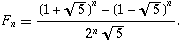SEARCH HOMEMath Central Quandaries & QueriesQuestion from Beth, a parent: You've answered a question for a Linda on Oct. 25, 1999 that has to do with a pet rabbit and stairs. (Working Backwards) We were wandering if there is a formula we can use to plug the numbers in? My 7th grader got that exact same problem. I've spent several hrs trying to come up with a formula. I've tried the combinations formula, the Pascal's triangle and the Permutations formula. It seems like it is something real close to those, but can't quite get anything to work. What kind of formula would work for finding the outcome of this problem? Thanks so much for your help. BethBeth,

this is the 'formula' for the Fibonacci numbers but it is not easy to implement:Penny

Beth wrote back

Thank you for sending the formula, but would you be so willing to plug the numbers in and show me how you got the answer to the 20 step problem? Also, does this formula have a name and what is it used primarily for?

Thanks again,
Beth

Beth,

I'm not sure what you're asking. The formula for the Fibonacci numbers is often called the Binet Formula. To use it for n = 20 is not really practical - we would use the formula to estimate the size of large Fibonacci numbers but for small n it is easier to use the recurrence F(n+1) = F(n)+F(n-1). The sequence starts off 1,2,2,3,5,8,13, ... . Each successive value is obtained from adding the two previous ones - for example the next number in the sequence is 8+13 = 21. This is the easiest way to get the 20th term.

PennyMath Central is supported by the University of Regina and The Pacific Institute for the Mathematical Sciences.